# Speed Tuning the Applet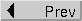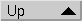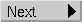Up to version V1.4, my Reversi applet was rather slow. In the process of developing V2.0, I not only improved the applet's playing strength, but also its speed. On this page, I'll discuss the techniques I used to achieve this speed-up. Some of the techniques are specific to programs having a structure similar to a strategy game program (i.e. recursive computations with a high branching factor), others apply equally to completely different programs.

Note: Although I use Java syntax to express code snippets here, I also tried to avoid idioms specific to the C/C++/Java world (e.g., the dreaded "++" pre- or post-increment operator). I hope even people who are not familiar with this syntax will understand the code snippets!

## Othello-Specific Optimizations

The techniques listed here actually are applicable to all programs that do recursive examination of a problem domain.

The single most important optimization is to compute things incrementally every time a move is made instead of computing it anew each time in the evaluation function. The reason is simple: the evaluation function is called far often than any other function in the applet.

Incrementally means that we compute a certain part of the evaluation function piece by piece, each time a move is made. Just as an example (and please note: this is not what I use as an evaluation function), if you want to compute the number of discs of a certain player that are on the board, you can either examine the whole board and count the discs:

 ```n_of_players_discs = 0; for (x = 1; x <= 8; x = x + 1) { for (y = 1; y <= 8; y = y + 1) { if (board[x][y] == player) n_of_players_discs = n_of_players_discs + 1; } }```

whenever you want to know that number, or you can update a current count of discs whenever a disc is set:

 ```/**** We assume a move (x, y) is being made by player 'player'. Also, we assume that 'n_of_discs' is set properly, i.e. in the beginning, it's initialized to n_of_discs[WHITE] = 2 and n_of_discs[BLACK] = 2. */ if (player == WHITE) opponent = BLACK; else opponent = WHITE; n_of_discs[player] = n_of_discs[player] + 1; /* The disc just set */ for (/* each disc to be turned by this move */) { /* flip disc */ n_of_discs[player] = n_of_discs[player] + 1; n_of_discs[opponent] = n_of_discs[opponent] - 1; }```

If you examine a game tree with e.g. 4 levels and approximately 10 possible moves on each level, you get about 104 calls to the evaluation function. In other words, in the first case, you'll examine the whole board 104 times just to count the number of discs of a player.

If you choose the second, incremental way, you'll update the count for each level once, i.e., most of the work is not done repeatedly, but just once. In the example, you'll update the count 104 times, but in doing so, you'll make far less array accesses than in the first case. The net result is that you'll do most of the work on the upper levels of the game tree only once instead of redoing it 10'000 times. Of course this saves time!

Now let's take a look at the components of my evaluation function that can be computed incrementally.

### The Edge Table

Of course the edge table is totally precomputed. It's the index in this table that interests us here. This index is the ternary number built from an edge configuration - an empty field counting as 0, a white disc as 1, and a black disc as 2. For instance, the edge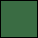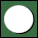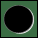would have the index 1 * 729 + 2 * 243 = 1215. Each field has a value determined by the disc (empty = 0, white = 1, black = 2) multiplied by successive powers of three. So the fields have the coefficients (from right to left) 1, 3, 9, 27, 81, 243, 729, and 2187. Simple addition of the values of all fields on an edge yields the index of the edge.

We can initialize these values with 0 (since at the beginning, an edge doesn't contain any discs), and the successively update these values whenever an edge move is played. This is much faster than scanning each edge in the evaluation function and then computing the index!

### Liberties

Before we'll treat the two other components of the evaluation function (mobility and potential mobility), we'll have to introduce an auxiliary measure: the liberties of a field. If you know Go, you already have a good idea of what this means: the liberty of a field is the number of empty fields around it.

This of course can be computed easily in an incremental way: we initialize a two-dimensional array properly:

 ```short liberties[][] = { { 3, 5, 5, 5, 5, 5, 5, 3 }, { 5, 8, 8, 8, 8, 8, 8, 5 }, { 5, 8, 7, 6, 6, 7, 8, 5 }, { 5, 8, 6, 5, 5, 6, 8, 5 }, { 5, 8, 6, 5, 5, 6, 8, 5 }, { 5, 8, 7, 6, 6, 7, 8, 5 }, { 5, 8, 8, 8, 8, 8, 8, 5 }, { 3, 5, 5, 5, 5, 5, 5, 3 } };```

and then we update all the field around a newly set field whenever we set a disc:

 ```/**** We assume a disc has just been set on (x, y). */ for (i = -1; i <= 1; i = i + 1) { for (j = -1; j <= 1; j = j + 1) { if (i != 0 || j != 0) { liberties[x+i][y+j] = liberties[x+i][y+j] - 1; } } }```

Computing liberties incrementally allows us to simplify the computation of the two measures that are really used in the evaluation function: mobility and potential mobility.

### Mobility

Mobility has been defined as the number of legal moves a player may make in a certain position. The question is therefore: how do we count these moves?

A naive approach is to simply scan the whole board. For each empty field we might look whether any discs of the opponent would be turned if we set there. If so, that field is a legal move. However, this approach necessitates that we scan the whole board (64 fields), and that for each field, we scan all diagonals to see whether we might flip some discs (at worst, 8 half-diagonals). That's quite a lot of work!

A better way is to update not only the indices for the edges incrementally, but to do so for all columns, rows, and even diagonals! This gives us 16 indices to maintain for the rows and colums, plus 30 for the diagonals. We then can save for each index the positions where either color may set, e.g., in above row, black may set on the leftmost field, while white can set on the fourth field from the left. With this arrangement, we only have to check 4 table entries (one for each diagonal, row and column a certain field lies on) to decide whether or not a move is legal. Again, since we can update these indices from move to move instead of having to recompute them wholly in the evaluation function, we'll save a lot of time.

We can even save a little more time if we observe that a possibly legal move can only be a currently empty field that is adjacent to some disc. In other words: only empty fields whose liberty is smaller than its initial value must be tested!

By the way, the world's strongest Othello program, Logistello, uses an approximation for mobility: if we can store in the table where a player may set, we can also store how many moves a player has on that row, column, or diagonal. Logistello then just sums up all these values for the whole board: 16 rows and columns and 22 diagonals on which moves are possible. This is even faster than scanning the whole board and testing for each field four table entries, but in the worst case, it may count a move four times instead of just once. It seems to me that this approximation is only good for large look-aheads such as Logistello can do (about 16 plys). For the shallow look-aheads that can be achieved by a Java applet, this approximation is too crude: it even completely misjudges positions sometimes, leading to very poor play. This effect is probably masked in Logistello because the deeper look-ahead may compensate for the weak evaluation. Also, this measure isn't Logistello's only evaluation criterion. Depending on its weight in the total evaluation, the effects of an imprecise approximation may be greater or smaller.

### Potential Mobility

There are basically three ways to estimate potential mobility:

• By counting the opponent's discs that are adjacent to an empty field,
• by counting all empty fields that are adjacent to at least one of the opponent's discs,
• or by summing the number of empty discs each opponent's disc touches.

The first was used up to V1.4 of my applet, computed each time anew in the evaluation function. Developing V2.0, I tried the two other possibilities, which both can be easily computed incremetally using the concept of liberties. My final conclusion was the following:

• Counting just the opponent's discs that are adjacent to an empty field tends to underestimate the potential mobility, because usually there are more empty fields adjacent to one of the opponent's discs. Also, it's not quite obvious to me how to compute this measure efficiently, i.e., incrementally.
• Counting the adjacent empty discs can be done incrementally, but doesn't make the applet play stronger.
• Summing up the number of adjacent discs also can be done incrementally, and although it overestimates potential mobility, using this measure resulted in a fairly strong program. This finding is supported by the statistical measurements Michael Buro made when he developed Logistello.

How to compute this measure? Well, given the liberties, we can simply sum the liberties of discs whenever we set a new disc:

 ```/**** We assume a move (x, y) is being made by player 'player'. */ if (player == WHITE) opponent = BLACK; else opponent = WHITE; /**** Handle the disc just set. */ potential[opponent] = potential[opponent] + liberties[x][y]; /**** Now update the liberties of the adjacent discs! */ update_liberties (x, y); for (/* each disc to be turned by this move */) { /* flip disc */ if (liberties[x][y] > 0) { potential[opponent] = potential[opponent] + liberties[x][y]; potential[player] = potential[player] - liberties[x][y]; } }```

That's it - more or less. There are a few details, such as my not regarding edge moves in this approximation of potential mobility, since this would overrate edge moves. But it is possible to compute a fairly good measure for potential mobility in an incremental way using the concept of liberty.

## General Speed-Tuning

In the first versions of my Reversi applet, I properly used object-oriented programming throughout. For instance, I had a class `Move`, and another class `Move_List` to record the possible moves in a given position. Also, when I set a move during look-ahead, I created a copy of the current board, on which I then set that move.

All very proper and clean, and all very slow.

Then I started to think. Why was my applet so slow? Well, let's see what happens. If we do a 4-ply look-ahead, and in each position we have about ten possible moves, we'll examine roughly 10'000 positions (assuming a simple minimax search, no alpha-beta pruning). In other words, we'll generate 10'000 objects for the various boards, and for the upper three levels of the game tree, we'll generate about 10'000 moves, collected in about 1'000 `Move_List`s.

This proliferation of objects puts a heavy burden on the memory management system. It becomes harder to find memory for a new object, the garbage collector runs more slowly (first, it must examine a lot of objects, and second, the storage for most of these objects may be reclaimed).

The solution was to make the game engine itself less object-oriented. Instead of creating new boards every time, I now use a stack of boards. These are created in the beginning, and there are enough of them to support the deepest look-ahead that will ever be done by my applet. The applet now re-uses these pre-allocated objects instead of always creating new ones.

This same method also was applied to the lists of possible moves: these have disappeared completely. Instead, I pre-allocate a stack of two arrays, one for the X-coordinates and one for the Y-coordinates of the moves.

(This is a case where I sorely miss the concept of a `struct` or `record` in Java - because even though Sun claims that these can be modelled by classes, the two concepts are not at all the same. With `struct`, I could allocate a stack of arrays of

 ```typedef struct { short X, Y; } Move;```

without having the tremendous overhead of a stack of array of objects of some class `Move`! As it is, I'm forced to use the archaic ways of Fortran (brrr!) and use "parallel" arrays to emulate an array of `struct`.)

Anyway, back to our moves: each list of moves is now stored as a simple sequence of coordinates in the arrays at the appropriate level of the stack. This saves another few thousand object creations and destructions!

To give you an idea of the importance of economizing objects in such an algorithm: using the idea of incremental computation, I was able to speed up the applet by maybe 30%. The other 70% of the speedup between V1.4 and V2.0 are due solely to this not creating that many objects! (V2.0 runs about 80% faster than V1.4: where V2.0 takes 20 seconds, V1.4 took about 2 minutes!)

## Conclusion

Two techniques were used to speed up the applet: incremental computation and creating as few objects as possible.

Incremental computation is equivalent to hoisting code out of nested loops. The more you can compute in the higher levels of the game tree, and the less work your evaluation function has to do, the faster your program will run.

Saving objects is always a good idea, but this technique should be employed with care. It's always better to design and implement a program properly at first, using objects where appropriate. Only if the resulting program doesn't meet the desired performance criteria, one should start to think about economizing objects. Still, it's a point to remember: careless use of objects may tremenduously slow down a program.

Ah yes, before I forget it, one word on the performance of alpha-beta pruning. I already stated that a simple unadorned minimax search will examine about 10'000 positions if we do a 4-ply look-ahead with a branching factor of 10. My applet uses the so-called "NegaScout" variant of alpha-beta pruning and examines in the worst case about 30% of these positions. But since already V1.4 employed the NegaScout algorithm, this doesn't count for the speed increase of V2.0.